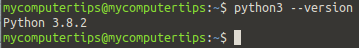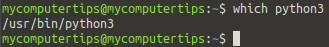Category: Python3

Viewed: 277 times

## How to create a basic Python3 script in Ubuntu and Ubuntu based distributions

Ubuntu and Ubuntu based distributions include Python3, so you can start programming without installing any additional packages.

In this example we will create a basic python script and run this through the terminal.

To start off, we can check what version of Python3 we have installed on our system, by issuing the following command in the terminal

python3 --version

As you can see from the screenshot, my system is running versionIn order to create a Python script we need to find the path to the Python interpreter on our system, so issue the following command in the terminal
which python3Next create a new file on your desktop and save as python.py
Next open the file and copy and paste the code below, noting the path to Python at the top of the script and save the file.

#!/usr/bin/python3
number1 = input("Enter your first number? ")
number2 = input("Enter your second number? ")
sum = int(number1) + int(number2)
print ("The answer is " +str(sum))

Now we need to make the file executable, so enter the following command in the terminal
chmod +x python.py

Now we can test the python script by entering the name of the file
./python.py

The script asks for two numbers via input, then adds the two numbers together.

Other Tips & Tutorials

Python3

How to create a basic Python3 script in Ubuntu and Ubuntu based distributions

Posted: 28th of April 2020

Views: 277